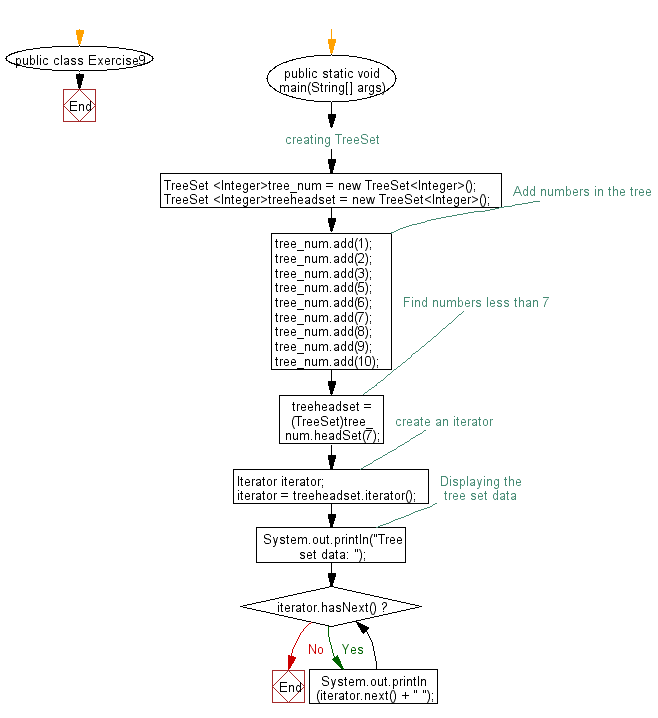﻿ Java: Find the numbers less than 7 in a tree set# Java Collection, TreeSet Exercises: Find the numbers less than 7 in a tree set

## Java Collection, TreeSet Exercises: Exercise-9 with Solution

Write a Java program to find the numbers less than 7 in a tree set.

Sample Solution:

Java Code:

``````import java.util.TreeSet;
import java.util.Iterator;

public class Exercise9 {
public static void main(String[] args) {
// creating TreeSet
TreeSet <Integer>tree_num = new TreeSet<Integer>();

// Add numbers in the tree

// Find numbers less than 7

// create an iterator
Iterator iterator;

//Displaying the tree set data
System.out.println("Tree set data: ");
while (iterator.hasNext()){
System.out.println(iterator.next() + " ");
}
}
}
``````

Sample Output:

```Note: Exercise9.java uses unchecked or unsafe operations.
Note: Recompile with -Xlint:unchecked for details.
Tree set data:
1
2
3
5
6 ```

Flowchart:Java Code Editor:

What is the difficulty level of this exercise?

Test your Programming skills with w3resource's quiz.

﻿

## Java: Tips of the Day

getEnumMap

Converts to enum to Map where key is the name and value is Enum itself.

```public static <E extends Enum<E>> Map<String, E> getEnumMap(final Class<E> enumClass) {
return Arrays.stream(enumClass.getEnumConstants())
.collect(Collectors.toMap(Enum::name, Function.identity()));
}
```

Ref: https://bit.ly/3xXcFZt